# Dual Nature of Radiation and Matter NCERT Exemplar Problems Solutions Physics

0
1862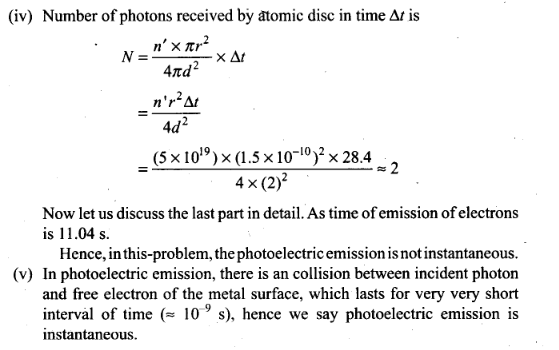## NCERT Exemplar Problems Class 12 Physics Chapter 11 Dual Nature of Radiation and Matter

Multiple Choice Questions (MCQs)

Question 1. A particle is dropped from a height H. The de-Broglie wavelength of the particle as a function of height is proportional to (a) H (b) H1/2 (c) H0 (d) H-1/2
Solution: (d)
Key concept: According to de-Broglie a moving material particle sometimes acts as a wave and sometimes as a particle.
The wave associated with a moving particle is called matter wave or de-Broglie wave and it propagates in the form of wave packets with group velocity. According to de-Broglie theory, the wavelength of de-Broglie wave is given by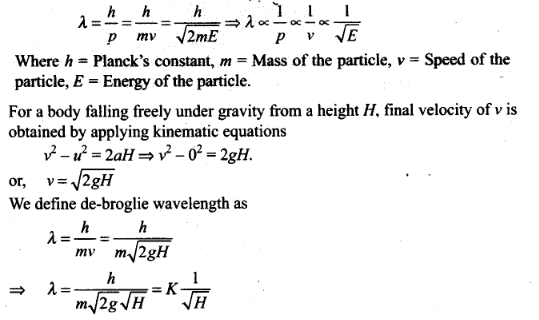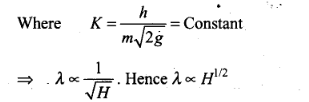Question 2. The wavelength of a photon needed to remove a proton from a nucleus which is bound to the nucleus with 1 MeV energy is nearly
a) 1.2 nm (b) 1.2 x 10-3 nm
(c) 1.2 x 10-6 nm (d). 1.2 x 10 nm
Solution: (b)
Key concept: According to Einstein’s quantum theory light propagates in the bundles (packets or quanta) of energy, each bundle being called a photon and possessing energy.
Energy of photon is given by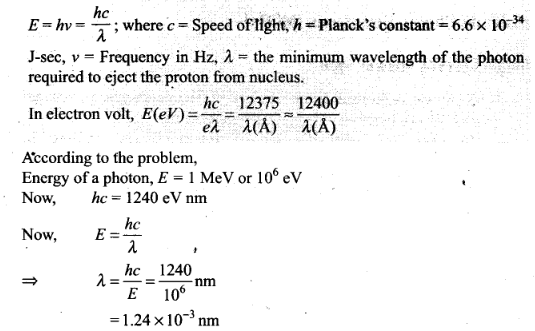Question 3. Consider a beam of electrons (each electron with energy E0) incident on a metal surface kept in an evacuated chamber. Then,
(a) no electrons will be emitted as only photons can emit electrons
(b) electrons can be emitted but all with an energy, E0
(c) electrons can be emitted with any energy, with a maximum of E0 – ɸ (ɸ is the work function)
(d) electron can be omitted with energy ,with a maximum of E0
Solution: (d) If a beam of electrons of having energy E0 is incident on a metal surface kept in an evacuated chamber.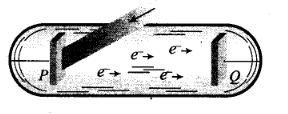The electrons can be emitted with maximum energy E0 (due to elastic collision) and With any energy less than E0, when part of incident energy of electron is used in liberating the electrons from the surface of metal.

Question 4. Consider the figure given below. Suppose the voltage applied to A is increased. The diffracted beam will have the maximum at a value of θ that (a) will be larger than the earlier value
(b) will be the same as the earlier value
(c) will be less than the earlier value
(d) will depend on the target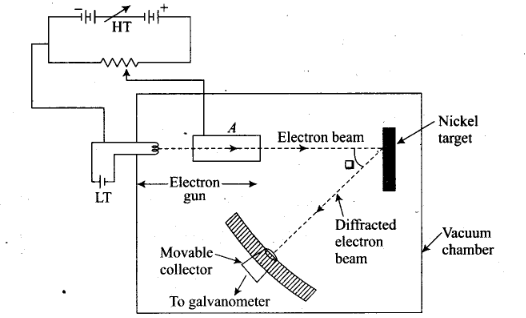Solution: (c)
Key concept: Davision and Germer Experiment:
1. It is used to study the scattering of electron from a solid or to verify the wave nature of electron. A beam of electrons emitted by an . electron gun is made to fall on nickel crystal cut along cubical axis at a particular angle. Ni crystal behaves like a three dimensional diffraction grating and it diffracts the electron beam obtained from electron gun.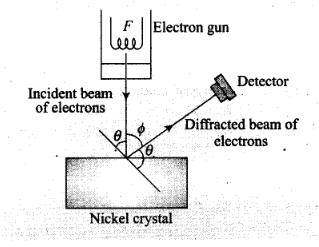2. The diffracted beam of electrons is received by the detector which can be positioned at any angle by rotating it .about the point of incidence. The energy of the incideni beam of electrons can also be varied by changing the applied voltage to the electron gun.
According to classical physics, the intensity of scattered beam of electrons at all scattering angle will be same but Davisson and Germer found that the intensity of scattered beam of electrons was not the same but different at different angles of scattering. It is maximum for diffracting angle 50° at 54 volt potential difference.
3. If the de-Broglie waves exist for electrons then these should be diffracted as X-rays. Using the Bragg’s formula 2d sinθ = nλ, we can determine the wavelength of these waves.
The de-Broglie wavelength associated with electron is where V is the applied voltage.
Using the Bragg’s formula we can determine the wavelength of these waves. If there is a maxima of the, diffracted electrons at an angle θ, then
2d sin θ = A (ii)
From Eq. (i), we note that if V is inversely proportional to the wavelength λ. i.e., V will increase with the decrease, in λ.
From Eq. (ii), we note that wavelength λ is directly proportional to sinθ and hence θ.
So, with the decrease in λ , θ will also decrease.
Thus, when the voltage applied to A is increased. The diffracted beam will have the maximum at a value of θ that will be less than the earlier value.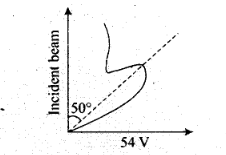4. A proton, a neutron, an electron and an a-particle have same energy. Then, their de-Broglie wavelengths compare as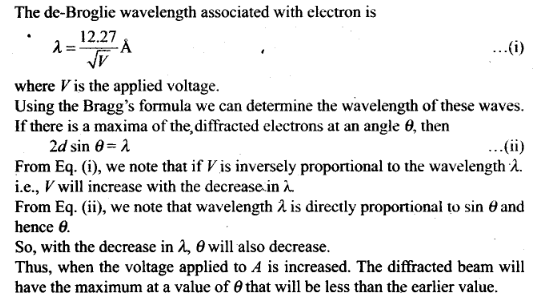Question 5. A proton, a neutron, an electron and an a-particle have same energy. Then, their de-Broglie wavelengths compare as (a) λp = λn > λe > λα (b) λα < λp = λn > λe
(c) λe< λp = λn> λα (d) λe = λp = λn = λα
Solution: (b)
Key concept:
• Matter Waves (de-Broglie Waves)
According to de-Broglie a moving material particle sometimes acts as a wave and sometimes as a particle.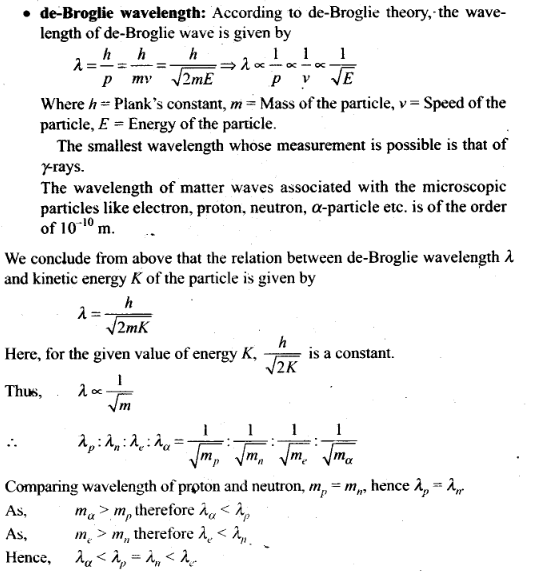Question 6. An electron is moving with an initial velocity v = v0i and is in a magnetic field B = B0j. Then, its de-Broglie wavelength
(a) remains constant
(b) increases with time
(c) decreases with time
(d) increases and decreases periodically
Solution: (a)
Key concept: If a particle is carrying a positive charge q and moving with a velocity v enters a magnetic field .5 then it experiences a force F which is given by the expression
F = q(v x B)=\$ F = qvB sin θ. As this force is perpendicular to v and B , so the magnitude of v will not change, i.e. momentum (p = mv) will remain constant in magnitude. Hence,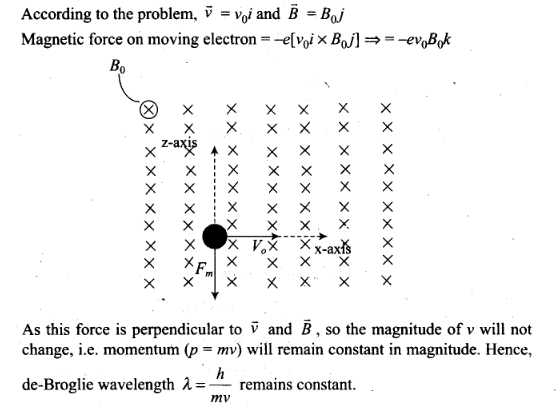Question 7. An electron (mass m) with an initial velocity v = v0i(v0 > 0) is in an electric field E =E0î (E0 = constant > 0). Its de-Broglie wavelength at time t is given by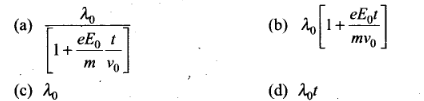Solution: (a)
Key concept: The wave associated with moving particle is called matter wave or de-Broglie wave and it propagates in the form of wave packets with group velocity. According to de-Broglie theory, the wavelength of de- Broglie wave is given by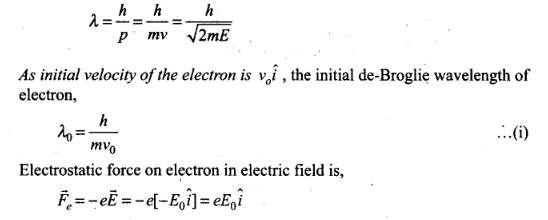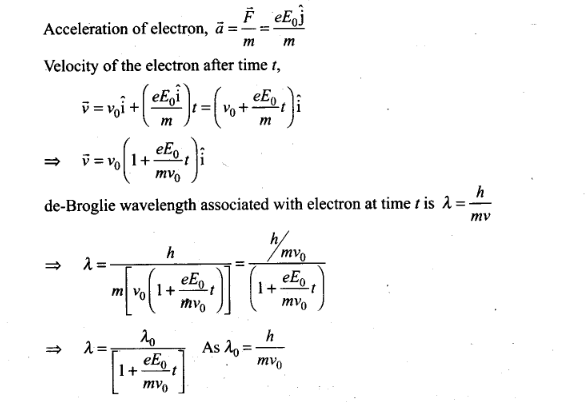Question 8. An electron (mass m) with an initial velocity v =v0î is in an electric field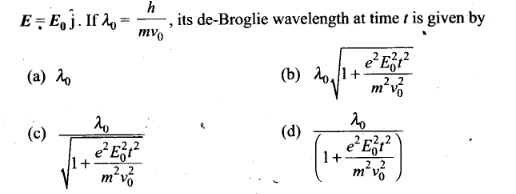Solution: (c) According to the problem de-Broglie wavelength of electron at time t=0.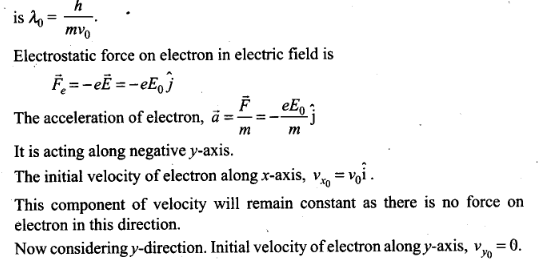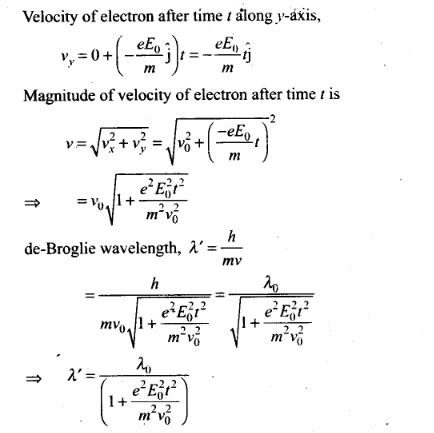One or More Than One Correct Answer Type

Question 9. Relativistic corrections become necessary when the expression for the kinetic
energy 1/2 mv2 , becomes comparable with mc2. where m is the mass of the
particle. At what de-Broglie wavelength, will relativistic corrections become important for an electron?
(a) A=10nm (b) A =10-1 nm (c) A=10-4 nm (d) A=10-6 nm
Solution: (c, d)
Key concept: De-Brogile or matter wave is independent of die charge on the material particle. It means, matter wave of de-Broglie wave is associated with every moving particle (whether charged or uncharged).
The de-Broglie wavelength at which relativistic corrections become important that the phase velocity of the matter waves can be greater than the speed of the light (3 x 108 m/s).
The wavelength of de-Broglie wave is given by
λ = h/p = h/mv
Here, h = 6.6 x 10-34 Js
and for electron, m = 9 x 10-31 kg
To approach these types of problem we use hit and trial method by picking up each option one by one.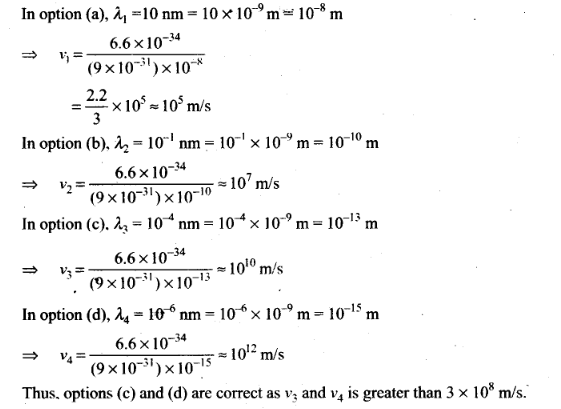Question 10. Two particles A1 and A2 of masses m1 , m2 ( m1> m2) have the same de-Broglie
wavelength. Then,
(a) their momenta are the same (b) their energies are the same
(c) energy of A1 is less than the energy of A2
(d) energy of A1 is more than the energy of A2
Solution: (a. c)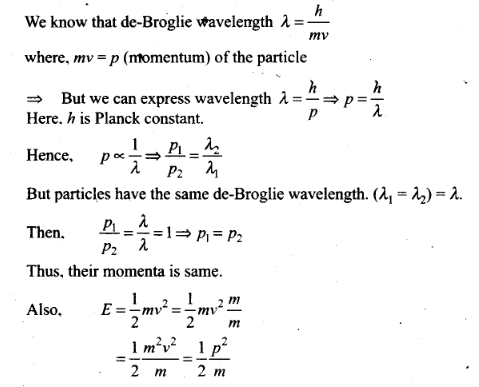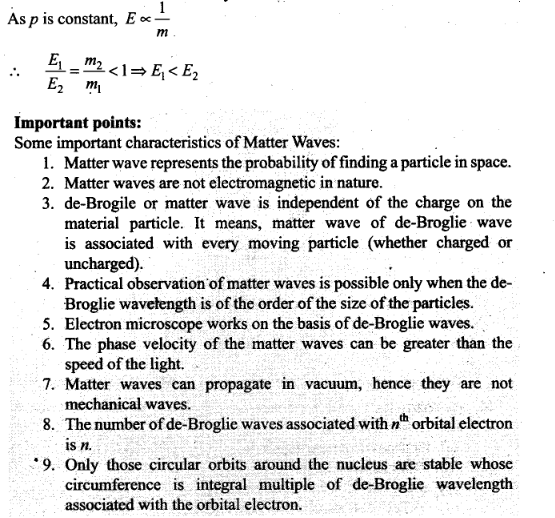Question 11. De-Broglie wavelength associated with uncharged particles: For Neutron efe-Broglie wavelength is given as ve=c/100.Then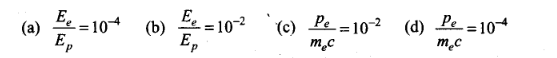Solution:(b,c)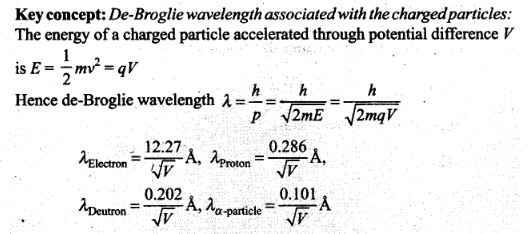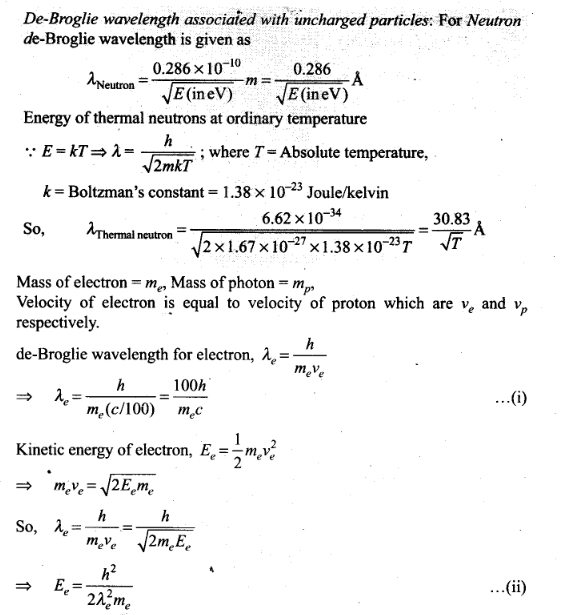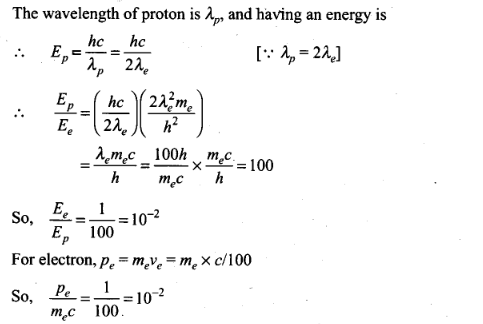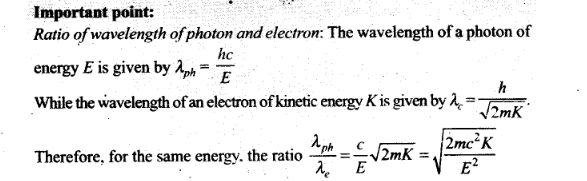Question 12. Photons absorbed in a matter are converted to heat. A source emitting v photon/sec of frequency v is used to convert 1 kg of ice at 0°C to water at 0°C. Then, the time T taken for the conversion
(a) decreases with increasing n. with v fixed
(b) decreases with n fixed, v increasing
(c) remains constant with n and v changing such that nv = constant
(d) increases when the product nv increases
Solution: (a, b. c).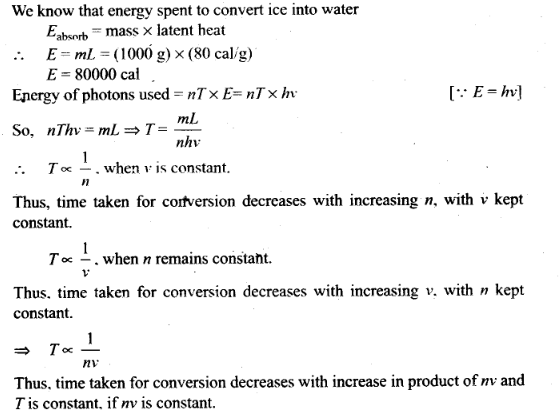Question 13. A particle moves in a closed orbit around the origin, due to a force which is directed towards the origin. The de-BrOglie wavelength of the particle varies cyclically between two values λ1, λ2 with λ1> λ2 Which of the following statements are true?
(a) The particle could be moving in a circular orbit with origin as centre
(b) The particle could be moving in an elliptic orbit with origin as its focus
(c) When the de-Broglie wavetength is λ1 the particle is nearer the origin than when its value is λ2
(d) When the de-Broglie wavelength is λ2 the particle is nearer the origin than when its value is λ1
Solution: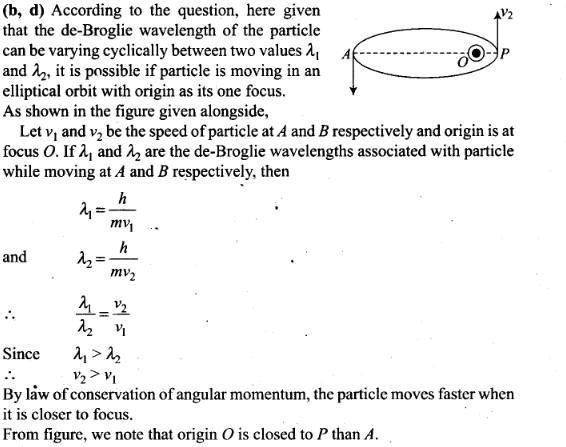Question 14. Aproton and an a-particle are accelerated, using the same potential difference. How are the de-Broglie wavelengths λp and λα related to each other?
Solution: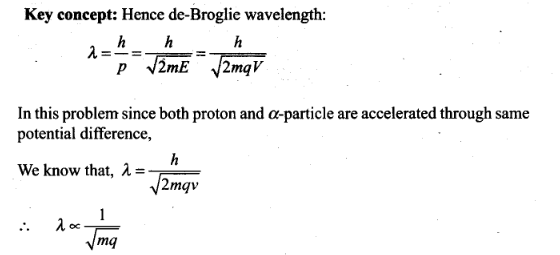Question 15. (i) In the explanation of photoeletric effect, we assume one photon of frequency v collides with an electron and transfers its energy. This leads . to the equation for the maximum energy Emax of the emitted electron as Emax = hv – ɸ0
where ɸ0 is the work function of the metal. If an electron absorbs 2 photons (each of frequency v), what will be the maximum energy for the emitted electron?
(ii) Why is this fact (two photon absorption) not taken into consideration in our discussion of the stopping potential?
Solution: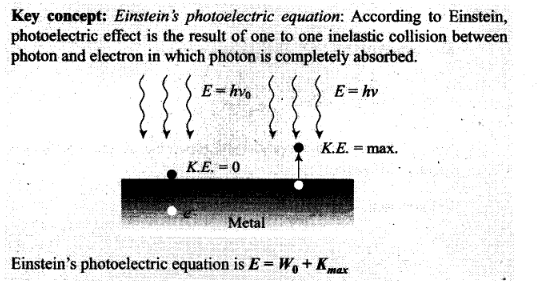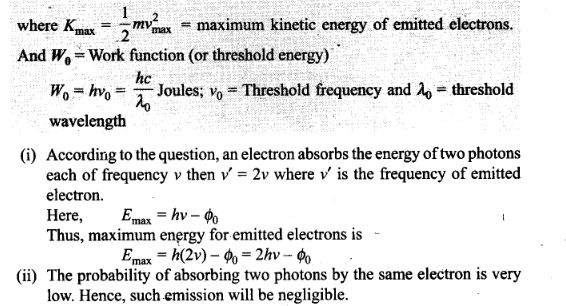Question 16. There are materials which absorb photons of shorter wavelength and emit photons of longer wavelength. Can there be stable substances which absorb photons of larger wavelength and emit light of shorter wavelength?
Solution: In the first case, when the materials which absorb photons of shorter wavelength has the energy of the incident photon on the material is high and the energy of emitted photon is low when it has a longer wavelength or in short we can say that energy given out is less than the energy supplied.
But in second case, the energy of the incident photon is low for the substances which has to absorb photons of larger wavelength and energy of emitted photon is high to emit light Of shorter wavelength. This means in this statement material has to supply the energy for the emission of photons.
But this is not possible for a stable substances.

Question 17. Do all the electrons that absorb a photon come out as photo electrons?
Solution:
Key concept: Photo-Electric Effect:
The photo-electtic effect is the emission of electrons (called photo-electrons when light strikes a surface. To escape from the surface, the electron must absorb enough energy from the incident radiation to overcome the attraction of positive ions in the material of the surface.
The photoelectric effect is based on the principle of conservation of energy.
1. Two conducting electrodes, the anode (Q) and cathode (P) are enclosed in an evacuated glass tube as shown on next page.
2. The battery or other source of potential difference creates an electric field in the direction from anode to cathode.
3. Light of certain wavelength or frequency falling on the surface of cathode causes a current in the external circuit called photoelectric current.
4. As potential difference increases, photoelectric current also increases till saturation is reached.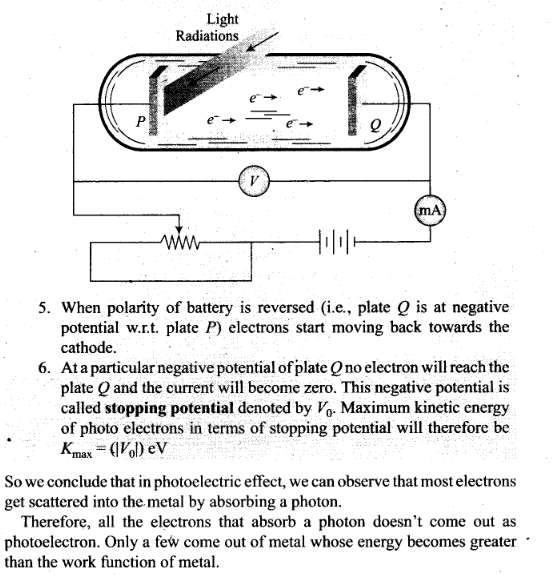Question 18. There are two sources of light, each emitting with a power of 100 W. One emits X-rays of wavelength 1 nm and the other visible light at 500 nm. Find the ratio of number of photons of X-rays to the photons of visible light of the given wavelength.
Solution:
Key concept: X-Rays:
1. X-rays were discovered by scientist Roentgen that is why they are also called Roentgen rays.
2. Roentgen discovered that when pressure inside a discharge tube is kept 10“3 mm of Hg and potential difference is kept 25 kV, then some unknown radiations (X-rays) are emitted by anode.
3. There are three essential requirements for the production of X-rays.
(i) A source of electron
(ii) An arrangement to accelerate the electrons
(iii) A target of suitable material of high atomic weight and high melting point on which these high speed electrons strike.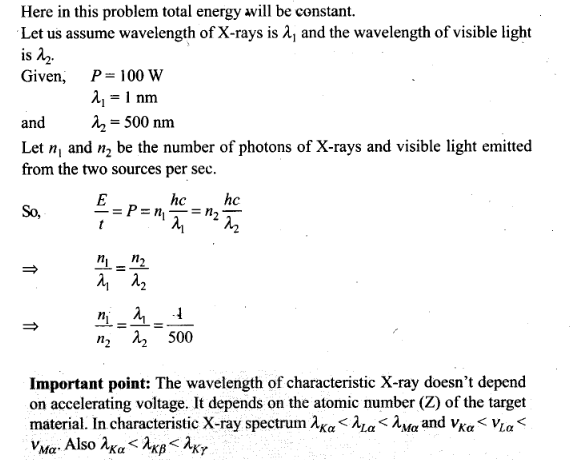Question 19. Consider a metal exposed to light of wavelength 600 nm. The maximum energy of the electron doubles when light of wavelength 400 nm is used. Find the work function in eV.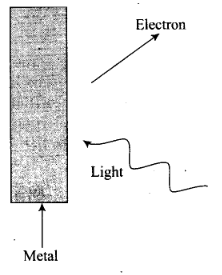Solution:The momentum of incident photon is transferred to the metal ,during photo electric emission.
At microscopic level ,atoms of a metal absorb the photon and its momentum is transferred mainly to the nucleus and electrons.The excited electron is emitted.Therefore,the conservation of momentum is to be considered as the momentum of incident photon transferred to the nucleus and electron.

Question 20.Consider a metal exposed to light the wavelength of 600 nm. The maximum energy of electron doubles when light of wavelength 400 nm is used.Find the work function in eV?
Solution: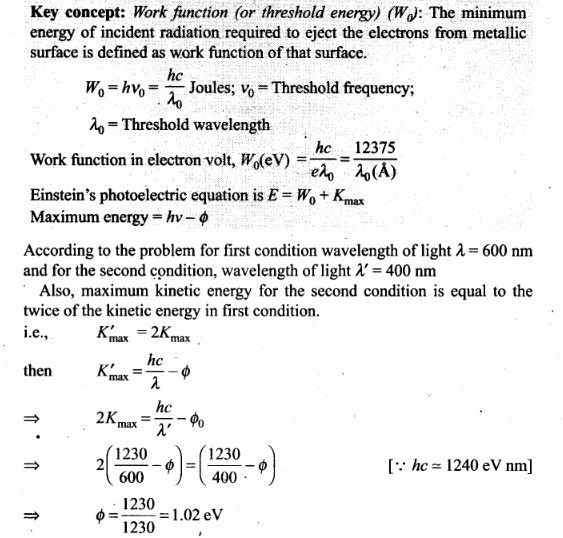Question 21. Assuming an electron is confined to a 1 nm wide region, find the uncertainty in momentum using Heisenberg uncertainty principle (∆x x∆p=h). You can assume the uncertainty in position ∆x as 1 nm. Assuming p = ∆p, find the energy of the electron in electron volts.
Solution: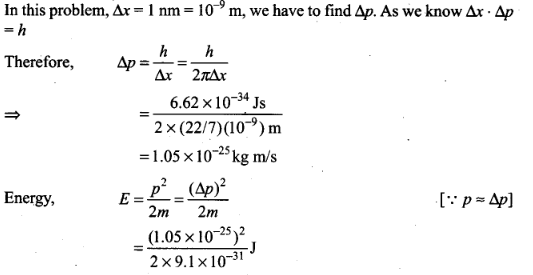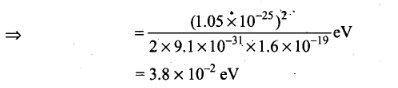Question 22. Two monochromatic beams A and B of equal intensity I, hit a screen. The number of photons hitting the screen by beam A is twice that by beam B. Then, what inference can you make about their frequencies?
Solution: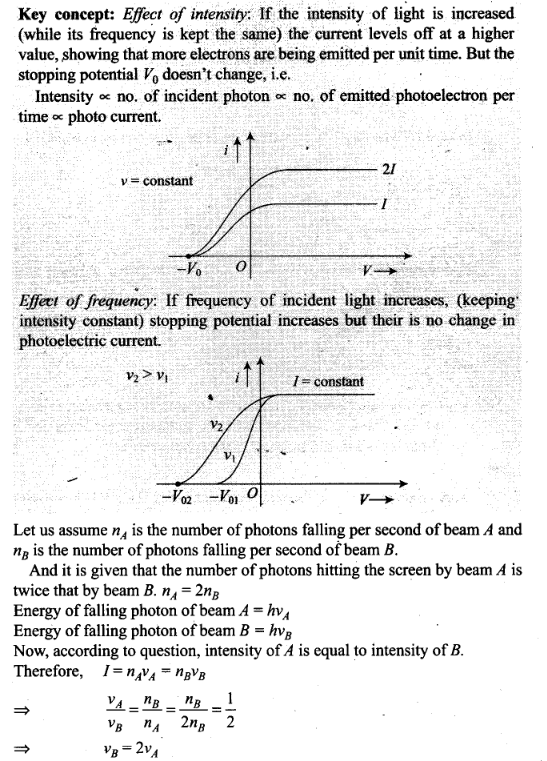Question 23. Two particles A and B of de-Broglie wavelengths A, and combine to form a particle C. The process conserves momentum. Find the de-Broglie wavelength of the particle C. (The motion is one-dimensional)
Solution: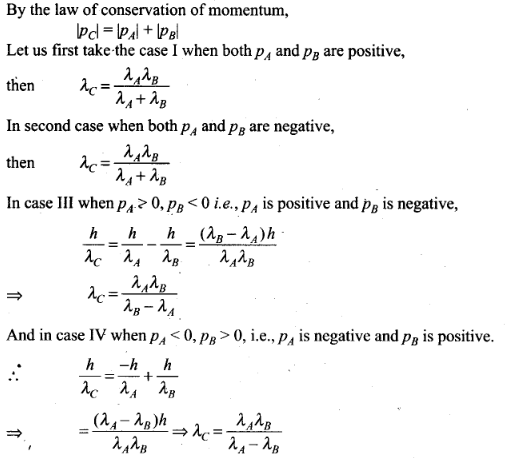Question 24. A neutron beam of energy E scatters from atoms on a surface with a spacing d = 0.1 nm. The first maximum intensity in the reflected beam occurs at θ= 30°. What is the kinetic energy E of the beam in eV?
Solution: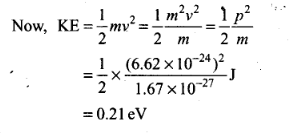Question 25. Consider a thin target (10-2 cm square, 10-3 m thickness) of sodium, which produces a photocurrent of 100 µA when a light of intensity 100 W/m2 (λ = 660 nm) falls on it. Find the probability that a photoelectron is produced when a photon strikes a sodium atom. [Take density of Na = 0.97 kg/m3]
Solution: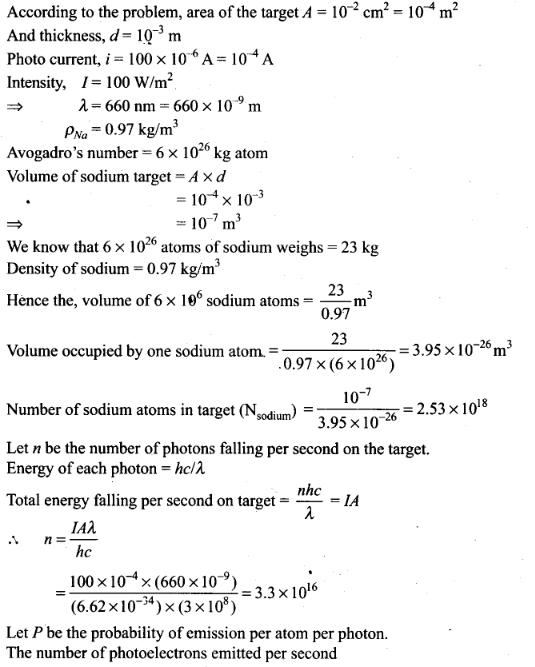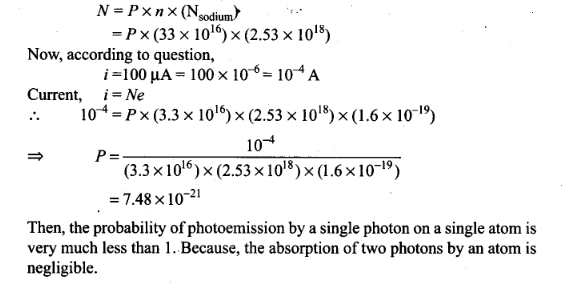Question 26. Consider an electron in front of metallic surface of a distance d.Assume the force of attraction by the plate is given as . Calculate work in taking the to an infinite distance from the plate .Taking d=0.1 nm. find the work done in electron volts?
Solution: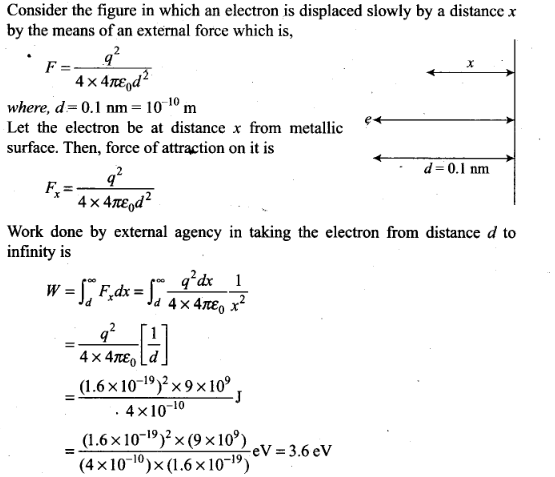Question 27. A student performs an experiment on photoelectric effect, using two materials A and B. A plot of Vstop versus v is given in figure.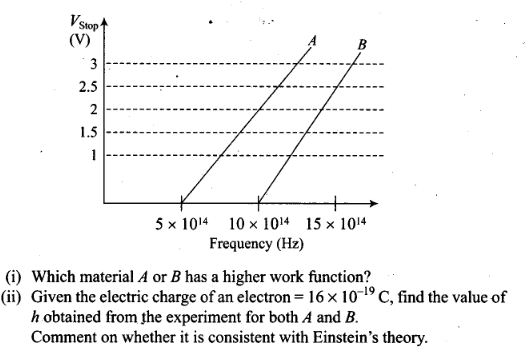Solution: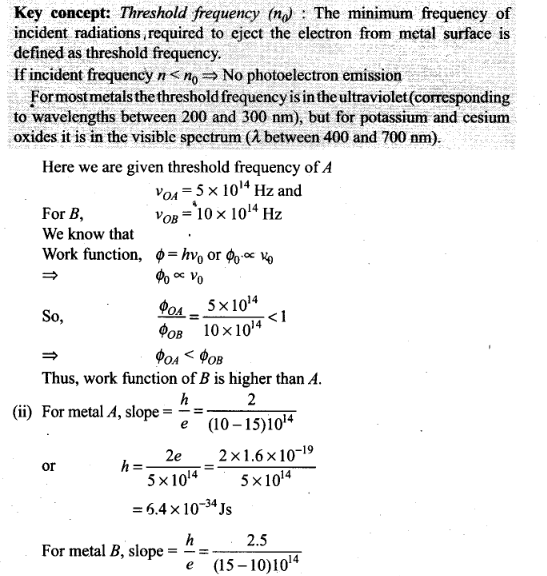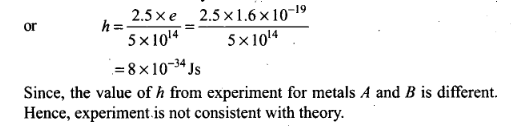Question 28. A particle A with a mass mA is moving with a velocity v and hits a particle B (mass mB) at rest (one dimensional motion). Find the change in the de-Broglie wavelength of the particle A. Treat the collision as elastic.
Solution: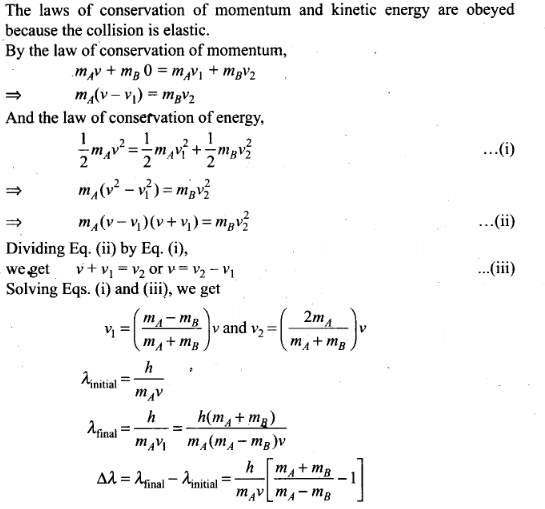Question 29. Consider a 20 W bulb emitting light of wavelength 5000 Å and shining on a metal surface kept at a distance 2 m. Assume that the metal surface has work function of 2 eV and that each atom on the metal surface can be treated as a circular disk of radius 1.5 Å.
(i) Estimate number of photons emitted by the bulb per second. [Assume no other losses]
(ii) Will there be photoelectric emission?
(iii) How much time would be required by the atomic disk to receive energy equal to work function (2 eV)?
(iv) How many photons would atomic disk receive within time duration calculated in (iii) above?
(v) Can you explain how photoelectric effect was observed instantaneously?
Solution: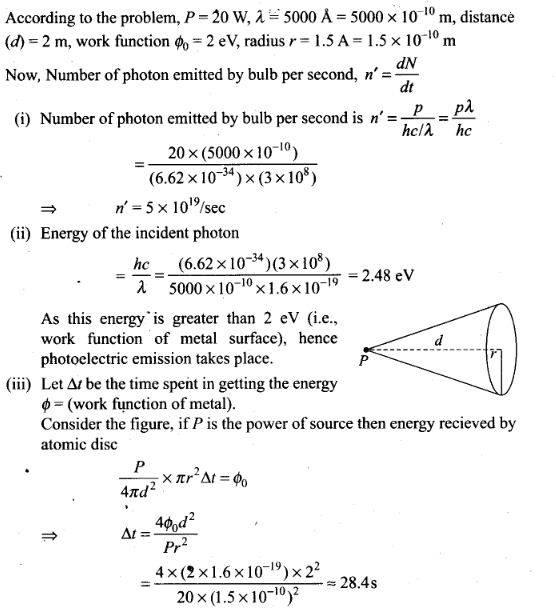Previous articleWave Optics NCERT Exemplar Problems Solutions Physics
Next articleAtoms NCERT Exemplar Problems Solutions Physics